# 扩展卡尔曼滤波EKF与多传感器融合

Extended Kalman Filter（扩展卡尔曼滤波）是卡尔曼滤波的非线性版本。在状态转移方程确定的情况下，EKF已经成为了非线性系统状态估计的事实标准。本文将简要介绍EKF，并介绍其在无人驾驶多传感器融合上的应用。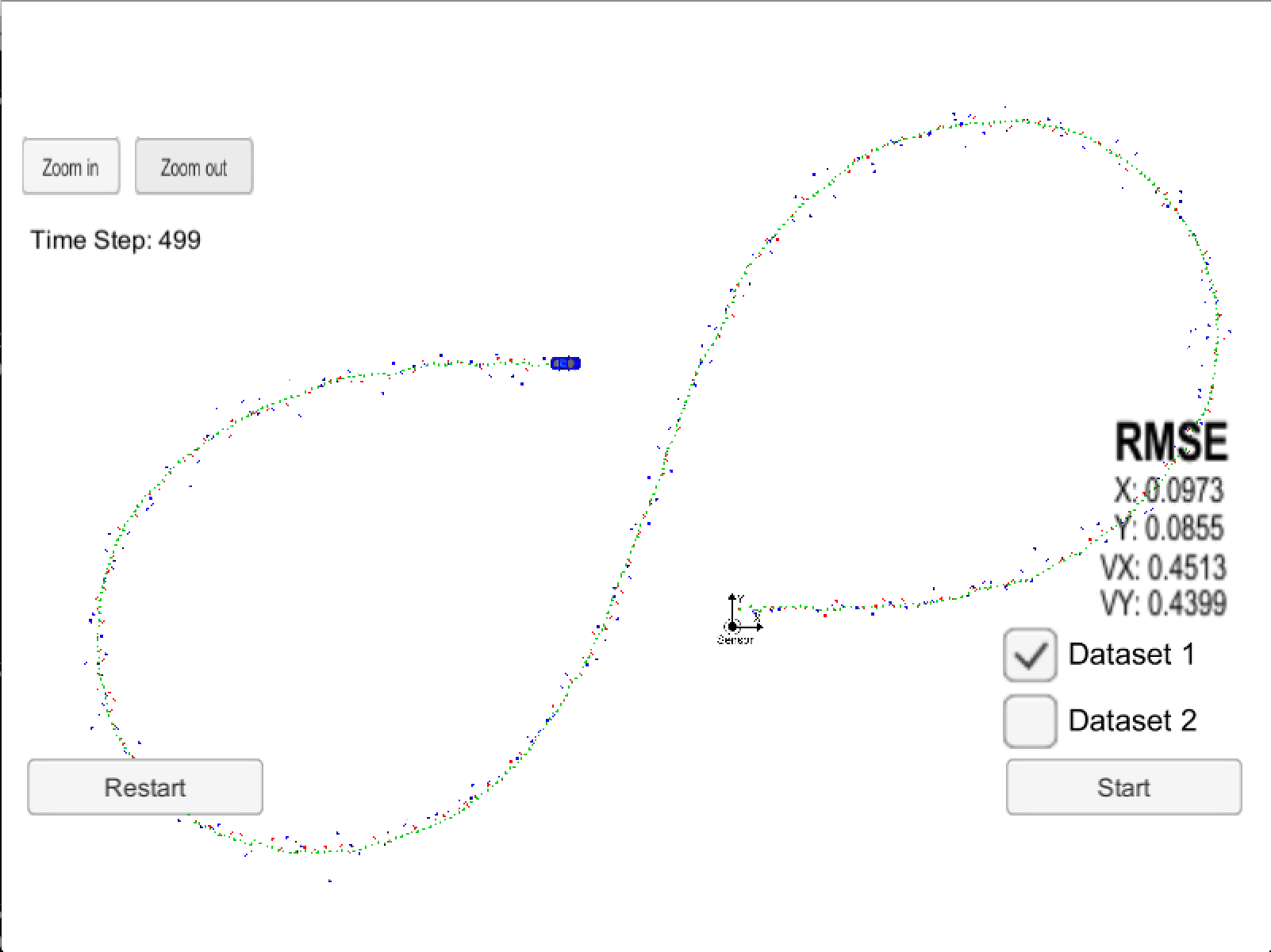# KF与EKF

KF与EKF的区别如下：

1. 预测未来：x=Fx+u$x'=Fx+u$x=f(x,u)$x'=f(x,u)$代替；其余F$F$Fj$F_j$代替。
2. 修正当下：将状态映射到测量的Hx$Hx'$h(x)$h(x')$代替；其余H$H$Hj$H_j$代替。

FjHj$F_j，H_j$计算方式如下：

Fjb=f(x,u)x=h(x)x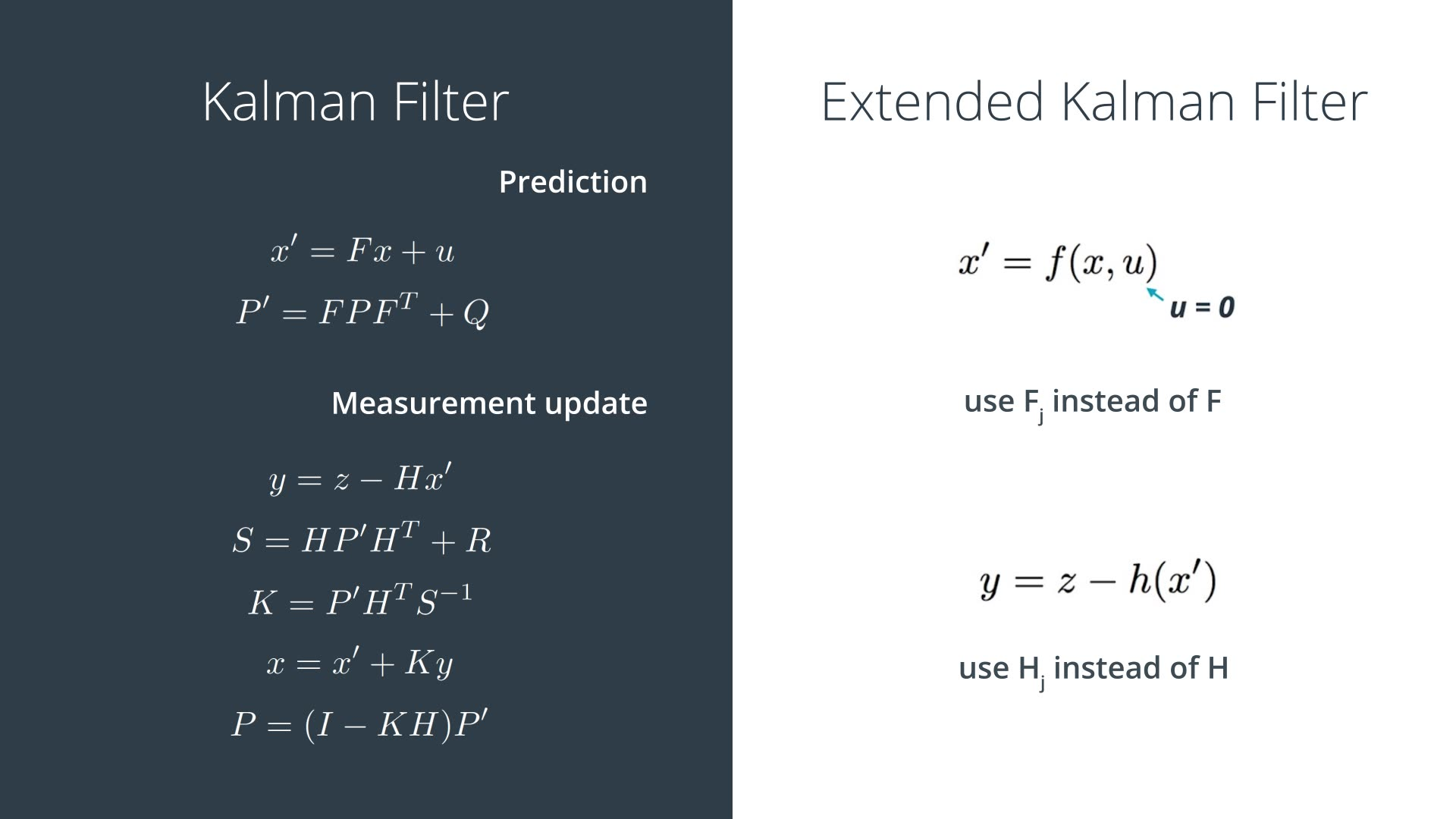## 为什么要用EKF

KF的假设之一就是高斯分布的x$x$预测后仍服从高斯分布，高斯分布的x$x$变换到测量空间后仍服从高斯分布。可是，假如FH$F、H$是非线性变换，那么上述条件则不成立。

## 将非线性系统线性化

f(x)f(μ)+f(μ)x(xμ)

T(x)f(a)+(xa)TDf(a)

# 多传感器融合

• lidar：笛卡尔坐标系。可检测到位置，没有速度信息。其测量值z=(px,py)$z=(p_x, p_y)$
• radar：极坐标系。可检测到距离，角度，速度信息，但是精度较低。其测量值z=(ρ,ϕ,ρ˙)$z=(\rho, \phi, \dot{\rho})$，图示如下。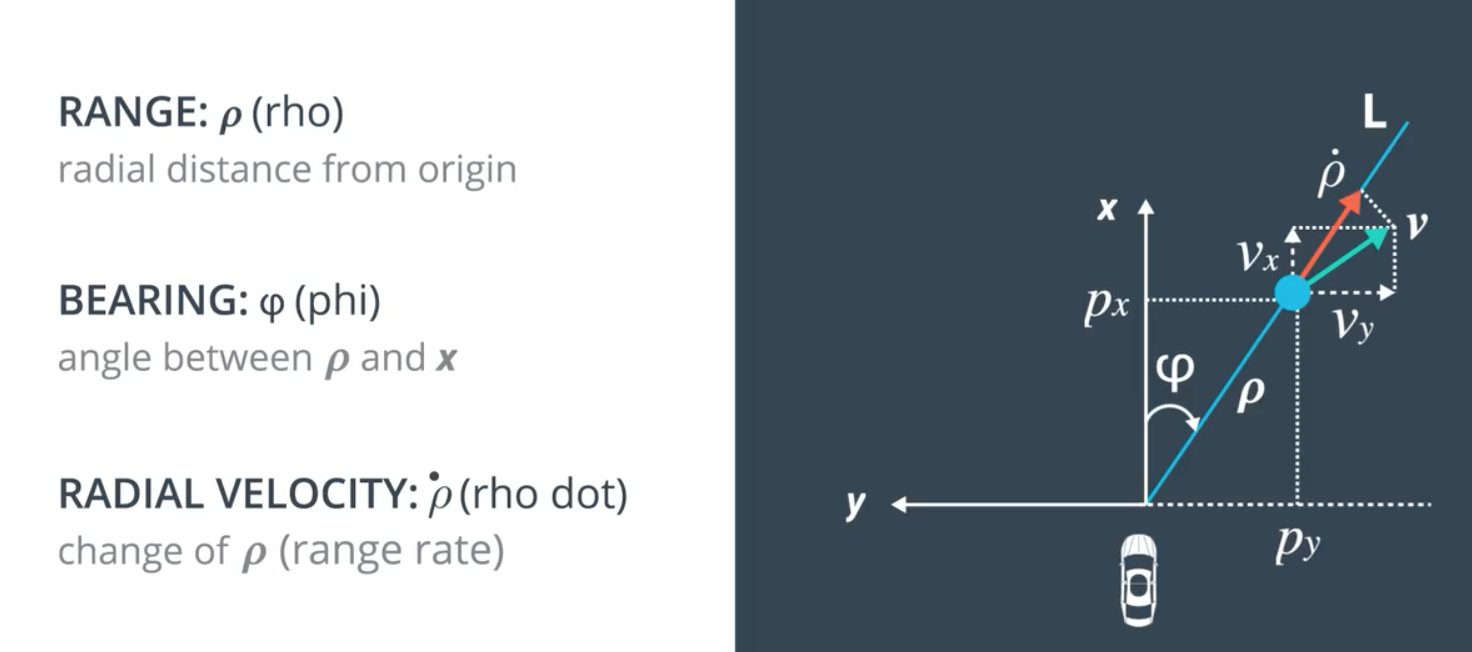## 传感器融合步骤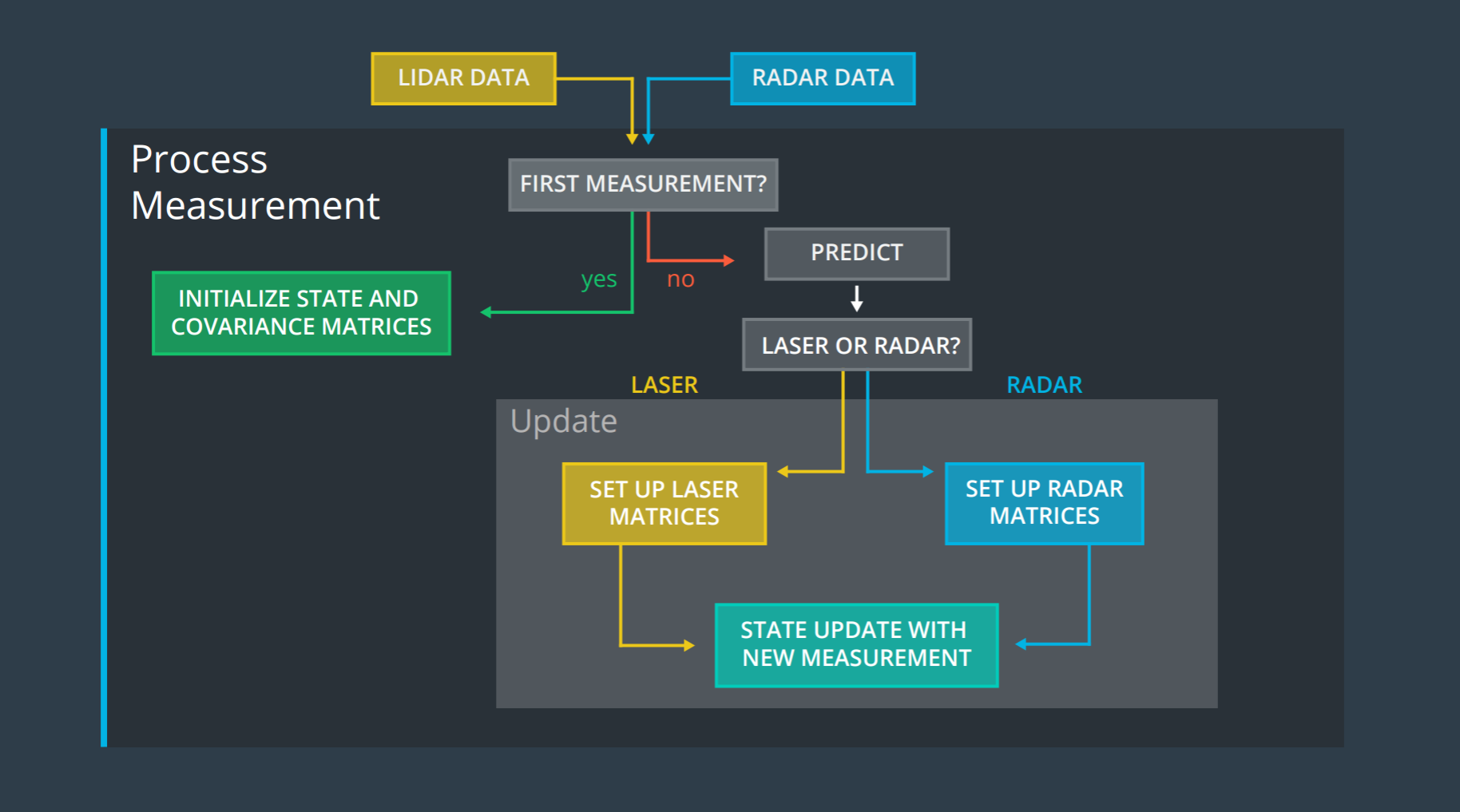1. 收到第一个测量值，对状态x$x$进行初始化。
2. 预测未来
3. 修正当下

## 初始化

pxpyvxvy=10000100[pxpy]

pxpyvxvy=ρcosϕρsinϕρ˙cosϕρ˙sinϕ

## 预测未来

xP=Fx=FPFT+Q

F$F$表明了系统的状态如何改变，这里仅考虑线性系统，F易得：

Fx=10000100dt0100dt01pxpyvxvy

P$P$表明了系统状态的不确定性程度，用x$x$的协方差表示，这里自己指定为：

P=1000010000100000001000

Q$Q$表明了x=Fx$x' = Fx$未能刻画的其他外界干扰。本例子使用线性模型，因此加速度变成了干扰项。x=Fx$x' = Fx$中未衡量的额外项目v$v$为：

v=axdt22aydt22axdtaydt=dt220dt00dt220dt[axay]=Ga

v$v$服从高斯分布N(0,Q)$N(0, Q)$

Q=E[vvT]=E[GaaTGT]=GE[aaT]GT=G[σ2ax00σ2ay]GT=dt44σ2ax0dt32σ2ax00dt44σ2ay0dt32σ2aydt32σ2ax0dt2σ2ax00dt32σ2ay0dt2σ2ay

## 修正当下

### lidar

lidar使用了KF。修正当下这里牵涉到的公式主要是：

ySKxP=zHx=HPHT+R=PHTS1=x+Ky=(IKH)P

H$H$表示了状态空间到测量空间的映射。

Hx=pxpyvxvy

R$R$表示了测量值的不确定度，一般由传感器的厂家提供，这里lidar参考如下：

Rlaser=[0.0225000.0225]

ySKxP=zf(x)=HjPHTj+R=PHTjS1=x+Ky=(IKHj)P

1. 状态空间到测量空间的非线性映射f(x)$f(x)$
2. 非线性映射线性化后的Jacob矩阵
3. radar的Rradar$R_{radar}$

f(x)=ρϕρ˙=p2x+p2yarctanpypxpxvx+pyvyp2x+p2y

Hj=f(x)x=ρpxϕpxρ˙pxρpyϕpyρ˙pyρvxϕvxρ˙vxρvyϕvyρ˙vy

R$R$表示了测量值的不确定度，一般由传感器的厂家提供，这里radar参考如下：

Rlaser=0.090000.00090000.09

# 传感器融合实例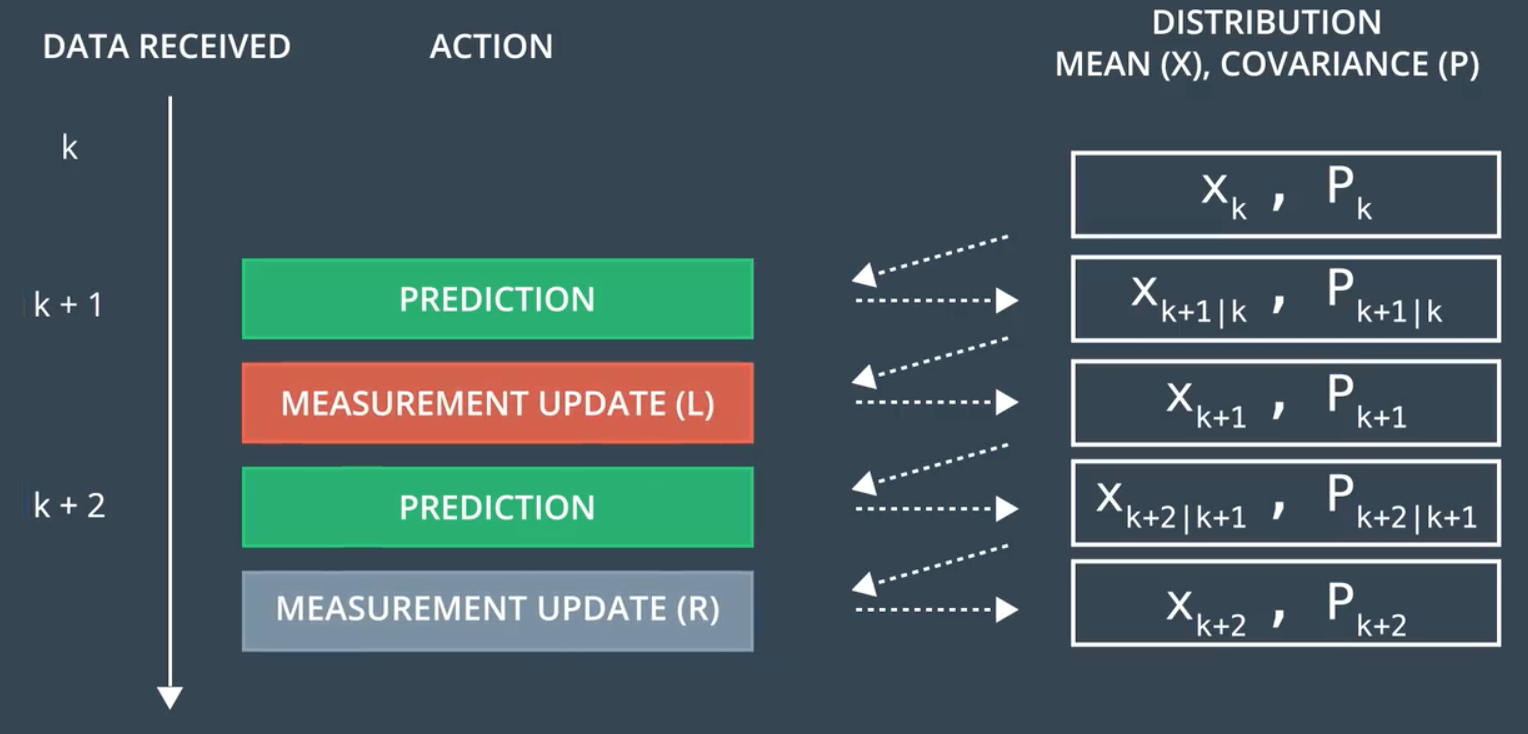06-19844

#### 【转】扩展卡尔曼滤波EKF与多传感器融合

04-065万+

#### 多传感器数据融合算法综述09-115万+

#### 卡尔曼滤波(KF)与扩展卡尔曼滤波(EKF)的一种理解思路及相应推导（1）

11-101万+

#### 卡尔曼滤波数据融合算法

08-059669

#### 惯性导航——扩展卡尔曼滤波（一）

04-26290

#### 无损卡尔曼滤波UKF 和 多传感器数据融合

01-18389

#### 多传感器融合之滤波（一）——卡尔曼滤波（KF）推导

04-062万+

#### 卡尔曼滤波系列——（二）扩展卡尔曼滤波

06-099988

#### 无人机姿态融合——EKF

05-042万+

#### MATLAB实现卡尔曼滤波器(KF、EKF)©️2020 CSDN 皮肤主题: 大白 设计师: CSDN官方博客点击重新获取扫码支付1.余额是钱包充值的虚拟货币，按照1:1的比例进行支付金额的抵扣。
2.余额无法直接购买下载，可以购买VIP、C币套餐、付费专栏及课程。余额充值